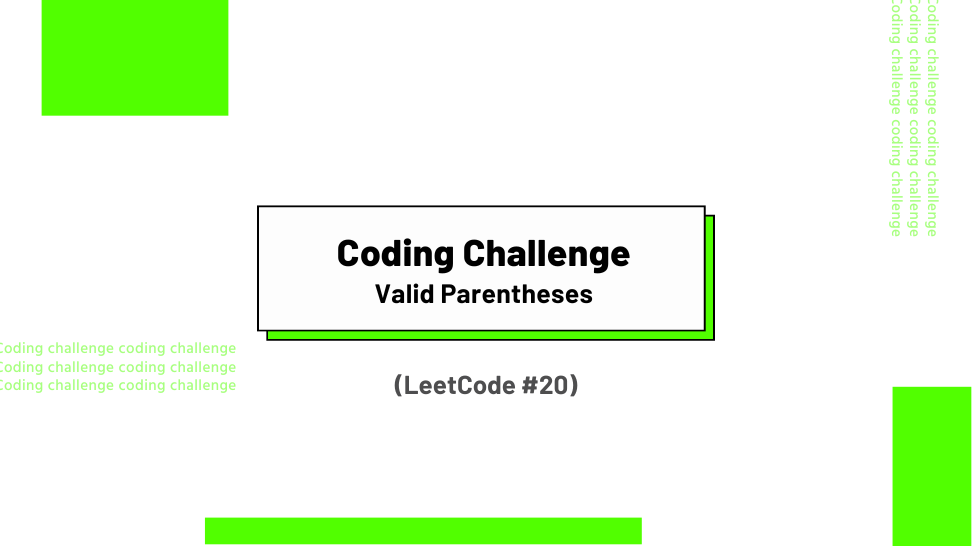## Challenge Statement

• Given a string s containing just the characters ‘(‘, ‘)’, ‘{‘, ‘}’, ‘[‘ and ‘]’, determine if the input string is valid.
• An input string is valid if:
• Open brackets must be closed by the same type of brackets.
• Open brackets must be closed in the correct order.
• This challenge corresponds to LeetCode #20.

### Constraints

• `1 <= s.length <= 104 `
• `s` consists of parentheses only ‘()[]{}’.

### Example 1:

Input: `s = "()"`

Output: `True`

### Example 2:

Input:` s = "()[]{}"`

Output: `True`

### Example 3:

Input: `s = "(]"`

Output: `False`

## Solution

Below is my solution and some test cases. The solution has a linear time complexity of O(n) and a linear space complexity O(n), where n is the length of the string s.